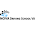## Monday, April 8, 2019

### G is for Global Fit Statistics

One of the challenges of Blogging A to Z is that the posts have to go in alphabetical order, even if it would make sense to start with a topic from the middle of the alphabet. It's more like creating the pieces of a puzzle, that could (and maybe should) be put together in a different order than they were created. But like I said, it's a challenge!

So now that we're on letter G, it's time to talk about a topic that I probably would have started with otherwise: what exactly is the Rasch measurement model? Yes, it is a model that allows you to order people according to their abilities (see letter A) and items according to their difficulties. But more that that, it's a prescriptive model of measurement - it contains within it the mathematical properties of a good measure (or at least, one definition of a good measure). This is how Rasch differs from Item Response Theory (IRT) models, though Rasch is often grouped into IRT despite its differences. You see, mathematically, Rasch is not very different from the IRT 1-parameter model, which focuses on item difficulty (and by extension, person ability). But philosophically, it is very different, because while Rasch is prescriptive, IRT is descriptive. If an IRT 1-parameter model doesn't adequately describe the data, you could just select a different IRT model. But Rasch says that the data must fit its model, and it gives you statistics to tell you how well it does. If your data don't fit the model, the deficiency is with the data (and your measure), not the model itself.

Note: This is outside of the scope of this blog series, but in IRT, the second parameter is item discrimination (how well the item differentiates between high and low ability candidates) and the third is the pseudo-guessing parameter (the likelihood you'd get an answer correct based on chance alone). The Rasch model assumes that the item discrimination for all items is 1.0 and does not make any corrections for potential guessing. You know how the SAT penalizes you for wrong answers? It's to discourage guessing. They don't want to you answering a question if you don't know the answer; a lucky guess is not a valid measure. What can I say, man? We psychometricians are a**holes.

When you use Rasch, you're saying that you have a good measure when it gives you data that fits the Rasch model. Poor fit to the Rasch model means you need to rework your measure - perhaps dropping items, collapsing response scales, or noting inconsistencies in person scores that mean their data might not be valid (and could be dropped from the analysis).

For Blogging A to Z 2017, I went through the alphabet of statistics, and for the letter G, I talked about goodness of fit. In Rasch, we look at our global fit statistics to see how well our data fit the prescribed Rasch model. If our data don't fit, we start looking at why and retooling our measure so it does.

The primary global fit statistic we should look at is the log-likelihood chi square statistic, which, as the name implies, is based on the chi square distribution. A significant chi-square statistic in this case means the data significantly differs from the model. Just like in structural equation model, it is a measure of absolute fit.

There are other fit statistics you can look at, such as the Akaike Information Criterion (AIC) and Schwarz Bayesian Information Criterion (BIC). These statistics are used for model comparison (relative fit), where you might test out different Rasch approaches to see what best describes the data (such as a Rating Scale Model versus a Partial Credit Model) or see if changes to the measure (like dropping items) results in better fit. These values are derived from the log-likehood statistic and either degrees of freedom for the AIC or number of non-extreme cases (in Rasch, extreme cases would be those that got every item right or every item wrong) for the BIC. (You can find details and the formulas for AIC and BIC here.)

BIC seems to be the preferred metric here, since it accounts for extreme cases; a measure with lots of extreme cases is not as informative as a measure with few extreme cases, so this metric can help you determine if dropping too easy or too difficult items improves your measure (it probably will, but this lets you quantify that).

1.2.3.